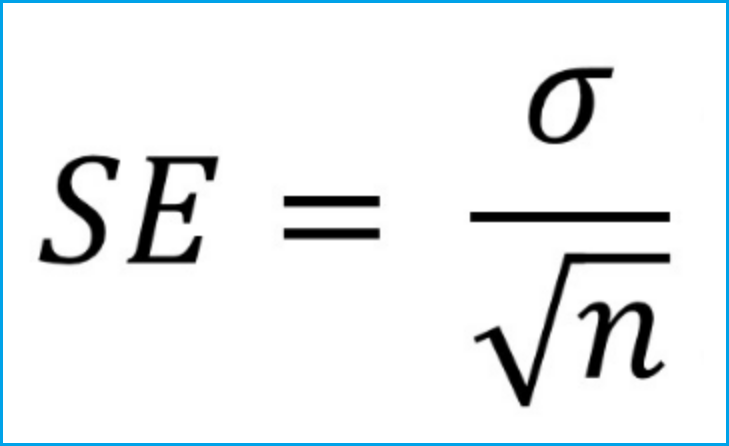# Standard Error in R (2 Examples)

This tutorial explains how to compute the standard error of the mean in the R programming language.

In the following, I’ll show you two different example codes for the computation of the standard error in R.

So without further ado, let’s dive in!

## Example 1: Compute Standard Error with User-Defined R Function

The basic installation of R does not provide a predefined function that calculates the standard error of the mean.

However, the formula of the standard error is quite simple and therefore we can easily create our own standard error function.

The standard error can be computed by dividing the standard deviation of our input by the square root of the length of our input…Figure 1: Formula of the Standard Error.

…and this formula can simply be reproduced with the sd, sqrt, and length functions in R:

`standard_error <- function(x) sd(x) / sqrt(length(x)) # Create own function`

Now, let’s apply this standard error function.

First, we need to create a numeric example vector in R…

`x <- c(4, - 1, 7, - 4, 6, 8, 10)                      # Example data`

…and then we can apply our previously created standard error function as follows:

```standard_error(x)                                     # Standard error own function
# 1.911298```

As you can see, the standard error of the mean of our example vector is 1.911298.

## Example 2: std.error Function [plotrix R Package]

You don’t want to create a function yourself? No problem! The plotrix add-on package includes the std.error function, which is also able to calculate the standard error of the mean.

First, let’s install and load the plotrix package:

```install.packages("plotrix")                           # Install plotrix R package

Now, we can apply the std.error R function as follows:

```std.error(x)                                          # Standard error with plotrix
# 1.911298```

The same result as in Example 1 – Looks good!

## Video, Further Resources & Summary

I have also published a video tutorial on this topic, so if you are still struggling with the code, watch the following video on my YouTube channel:

Please accept YouTube cookies to play this video. By accepting you will be accessing content from YouTube, a service provided by an external third party.If you accept this notice, your choice will be saved and the page will refresh.

The computation of the standard error of the mean is straight forward in R. However, the theoretical statistical concept might be more difficult to understand.

In case you want to know more about the concept of the standard error, you may have a look at the following YouTube video of the Khan Academy:

Please accept YouTube cookies to play this video. By accepting you will be accessing content from YouTube, a service provided by an external third party.If you accept this notice, your choice will be saved and the page will refresh.

In addition, you might be interested to read some of the other R tutorials of this homepage:

In conclusion: this article explained how to compute the standard error of the mean in the R programming language. If you have further comments or questions, please let me know in the comments below.

Subscribe to the Statistics Globe Newsletter